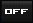View Single Post11-29-2009, 11:04 PM #9 THE eXchanger Avalon Senior Member   Join Date: Aug 2008 Location: Spiritual eXplorer-Canada Posts: 4,915Re: THE TRUTH about Earth Changes & ETs -- They are us, we are them Sal, and, Susan crossed paths online, in a group called www.soulwise.net back in 2002 - a year of an energetic "22" (2+0+0+2=22) Although this year, could be said, to be a "4" You do NOT reduce master numbers -- when 11 or 22 show up -- do NOT reduce them 1, 3, are both simple numbers 2 is the first complex number, where 2 simple numbers are added together (1+1=2) 3 is the first number, where one simple number + one complex number, are added together (1+2=3) or, where 3 simple numbers 1+1+1 = 3 or, where 1 simple number 1 is multiplied by 3 = 3 This is called a Trine / or a Trinity 4 - is the first number, where 2 complex numbers are added together or, 1 simple number, is addeded with 1 complex number (1+3=4) or, 2 complex numbers, number 2 are multiplied by 2 = 4 5 - is can be complex, so, we will discuss it, on it's own (later) 6 - is the second double of 2 complex numbers (3 x 2) or 6 - is the sum, of the first simple number, the complex number (5) + 1 = 6 added together 7- (i'll get into that later) - as, it can be added several ways 1+6 2+5 3+4 4+3 5+2 6+2 8- is the third double, where 2 x 2 x 2 = 8 albeit, it can also be factered 1+7 2+6 3+5 4+4 5+3 6+2 7+1 9 - is a triplicate of the multiplication of 3, the 2nd, simple number 3 X 3 or, can be factered as; 1+8 2+7 3+6 4+5 5+4 6+3 7+2 8+1 10 - 5 x 2 or 1+9 2+8 3+7 4+6 5+5 6+4 7+3 8+2 9+1 11 - is the first master number 12 - is the second master number and, can also be factored as: 4 times 3 , or 3 times 4 2 times 6, or 6 times, 2 etc., (and, has an assortment of factors-too numerous to mention here) 13 - is the 3rd master number 22 - is the 4rd master number 33- is the 5th master number (We will expand this, a little later) as, it is important to know (most here, probably already know this) Love/Susan The eXchanger Last edited by THE eXchanger; 12-01-2009 at 03:15 AM.